# Texas Go Math Kindergarten Module 6 Assessment Answer Key

Refer to our Texas Go Math Kindergarten Answer Key Pdf to score good marks in the exams. Test yourself by practicing the problems from Texas Go Math Kindergarten Module 6 Assessment Answer Key.

## Texas Go Math Kindergarten Module 6 Assessment Answer Key

Concepts and Skills

DIRECTIONS: 1. Count the dots of each color in the ten frames. Write the numbers. Trace and write the numbers in order as you count forward from the dashed number. (TEKS K.2.C) 2. Make a cube train with 6 cubes. Make a cube train with 4 cubes. Compare the cube trains by matching. Draw the cube trains. Write how many. Circle the cube train with more cubes. (TEKS K.2.G)

Question 1.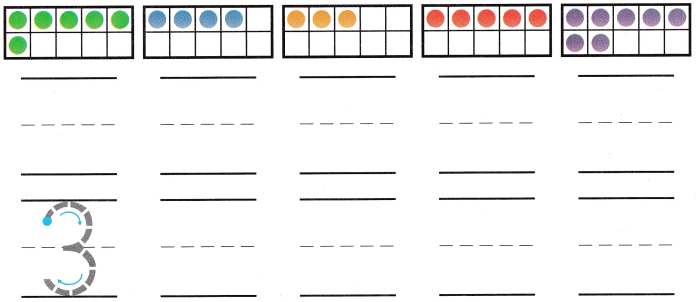Explanation:
Number of dots = 6, 4, 3, 5, 7.
Order of numbers:
3, 4, 5, 6, 7.

Question 2.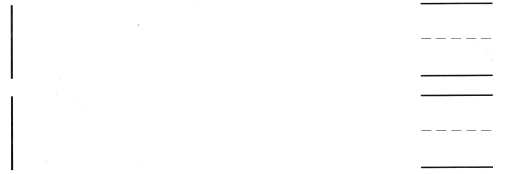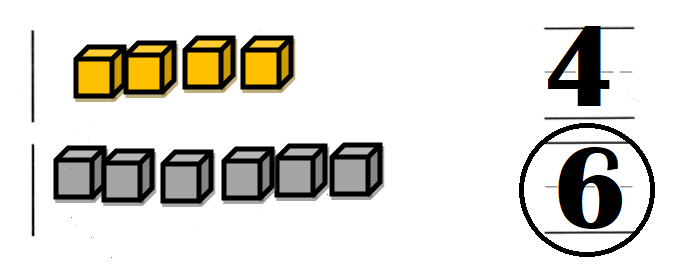Explanation:
Number of cubes:
4 and 6.
4 < 6.
6 > 4.

DIRECTIONS: 3. Write the number of objects in each set. Circle the greater number. (TEKS K.2.G) 4. Look at the number. Write the number that is one less. Write the number that is one more. (TEKS K.2.F) 5. Draw a set that has one fewer apple. 6. Look at the marbles. Draw a set that has fewer. (TEKS K.2.E) 7-8. Choose the correct answer. Which number is greater? (TEKS K.2.F)

Question 3.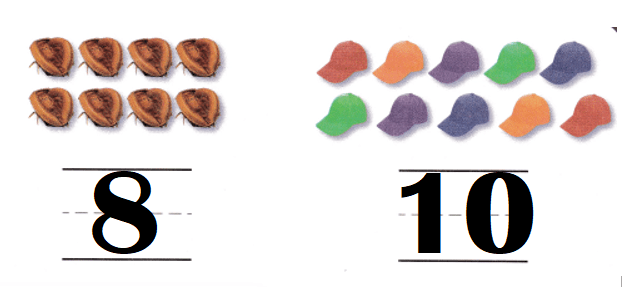Explanation:
Number of insects = 8.
Number of hats = 10.
=> 8 < 10.
10 > 8.

Question 4.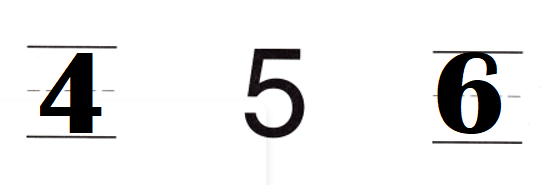Explanation:
One less than 5 = 5 – 1 = 4.
One more than 5 = 5 + 1 = 6.

Question 5.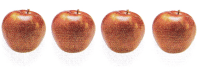Explanation:
Number of apples = 4.
One less than apples = 4 – 1 = 3

Question 6.Explanation:
Number of marbles = 9.
One less than 9 = 9 – 1 = 8.

Question 7.
Texas Test Prep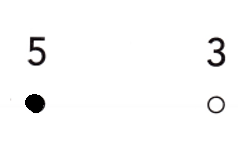Explanation:
Number 5 is < than number 3.

Question 8.